# FIT

## Introduction

FIT is a software package for integration of transcriptome data of samples in the field and meteorological data by modeling their relation. This software defines a statistical model of transcriptomes and provides an efficient method for training the model and for transcriptome prediction of unsequenced samples. Given the attributes of samples and meteorological data, this software predicts expression of a gene as $\hat{\boldsymbol s}=\beta_0 + \boldsymbol X \boldsymbol \beta,$ where $$\hat{\boldsymbol s}$$ is the predictions of $$\log_2$$-transformed values of the normalized expression levels, and $$\boldsymbol \beta_0$$ is a constant. Design matrix $$\boldsymbol X$$ consists of the plant’s age and the genotype of the samples, the circadian clock, the response to environmental stimuli, and the interactions between the age and the clock and the age and the environmental response.

The plant’s age is the vector of the number of days after transplanting; it is scaled to have the mean of $$0$$ and standard deviation of $$1$$. The circadian clock is represented by the linear combination of the cosine and sine curves with a $$24~\mathrm{h}$$ period. The response to environmental stimuli is the cumulative sum of nonlinearly transformed environmental stimuli during a given time period.

The model is specified by a set of regression coefficients and other parameters that are used for transformation of meteorological data into the input variables for regression of the expectation values for the gene expression. Optimization of regression coefficients $$\beta_0$$ and $$\boldsymbol \beta$$ and variable selection are simultaneously performed using an adaptive group lasso (Wang and Leng (2008)). Thus, this software explores the regression coefficients minimizing following cost function: $\left(\hat{\boldsymbol s} - \boldsymbol s\right)^T \left(\hat{\boldsymbol s} - \boldsymbol s\right) +\lambda\left(\sum_{k\in \{d, r, dr, n\}}\zeta_k|\beta_k|+\zeta_{c}\sqrt{\beta_{cos}^2+\beta_{sin}^2}+\zeta_{dc}\sqrt{\beta_{dcos}^2+\beta_{dsin}^2}\right),$ where $$\boldsymbol s$$ is the observed $$\log_2$$-transformed values of the normalized expressions, $$\lambda$$ is the regularization parameter, and $$\zeta_j$$ is the adaptive weight for penalizing each covariate. The values of parameters $$\lambda$$ and $$\zeta_j$$ are automatically selected in the software. Regression coefficients, $$\beta_d$$, $$\beta_r$$, $$\beta_{dr}$$, $$\beta_{n}$$, $$\beta_{cos}$$, $$\beta_{sin}$$, $$\beta_{dcos}$$, and $$\beta_{dsin}$$ correspond to the plant’s age, the response to environmental stimuli, their interaction, the genotype, the cosine and sine components of the circadian clock, and those of the interaction between the age and the circadian clock. Parameters related to the transformation of meteorological data are optimized by means of the Nelder-Mead algorithm (Nelder and Mead (1965)).

More details of the model is given in later sections or see the article by Iwayama et al. (2017).

## Installation

FIT can be easily installed from CRAN by typing the following command in an R session:

install.packages('FIT')

To install on Windows, the INSTALL_opts option is required as follows:

install.packages("FIT", INSTALL_opts = "--no-multiarch")

## Getting Started

### Prepare synthetic data

As an example, we generate synthetic RNA-Seq data. First, we load the meteorological data included in FIT package.

train.weather.file <- system.file('extdata', 'train.weather', package='FIT')
head(weather)
##    time temperature
## 10   10        14.5
## 20   20        14.7
## 30   30        14.3
## 40   40        13.9
## 50   50        14.0
## 60   60        14.0

This data contains temperature and radiation measured every 10 m and time column is the offset in minutes from May 1st, 2008 at 00:00:00.

weather.start.date <- as.POSIXct("2008-05-01")

In this example, we assume the following situation. Rice plants were transplanted into a paddy field on June 1 and two samples were collected every week from June 12 to September 18, 2008 for 24 h at each time at intervals of 4~$$h$$.

transplant.date <- as.POSIXct("2008-06-01")

sampling.date <- c(
as.POSIXct("2008-06-12") + as.difftime(rep(seq(0, 20, 4), each=2), unit="hours"),
as.POSIXct("2008-06-19") + as.difftime(rep(seq(0, 20, 4), each=2), unit="hours"),
as.POSIXct("2008-06-26") + as.difftime(rep(seq(0, 20, 4), each=2), unit="hours"),
as.POSIXct("2008-07-03") + as.difftime(rep(seq(0, 20, 4), each=2), unit="hours"),
as.POSIXct("2008-07-10") + as.difftime(rep(seq(0, 20, 4), each=2), unit="hours"),
as.POSIXct("2008-07-17") + as.difftime(rep(seq(0, 20, 4), each=2), unit="hours"),
as.POSIXct("2008-07-24") + as.difftime(rep(seq(0, 20, 4), each=2), unit="hours"),
as.POSIXct("2008-07-31") + as.difftime(rep(seq(0, 20, 4), each=2), unit="hours"),
as.POSIXct("2008-08-07") + as.difftime(rep(seq(0, 20, 4), each=2), unit="hours"),
as.POSIXct("2008-08-14") + as.difftime(rep(seq(0, 20, 4), each=2), unit="hours"),
as.POSIXct("2008-08-21") + as.difftime(rep(seq(0, 20, 4), each=2), unit="hours"),
as.POSIXct("2008-08-28") + as.difftime(rep(seq(0, 20, 4), each=2), unit="hours"),
as.POSIXct("2008-09-04") + as.difftime(rep(seq(0, 20, 4), each=2), unit="hours"),
as.POSIXct("2008-09-11") + as.difftime(rep(seq(0, 20, 4), each=2), unit="hours"),
as.POSIXct("2008-09-18") + as.difftime(rep(seq(0, 20, 4), each=2), unit="hours")
)
sampling.time <- as.numeric(sampling.date - weather.start.date, unit="mins")

Here, we consider five types of genes. The mean expression values of these genes vary according to the circadian clock, the response to environmental stimuli, and the plant’s age at the times of sampling. We calculate the mean expression values as below.

sample.n <- length(sampling.time)
mean.expression <- matrix(c(5, 5, 5, 4, 7), sample.n, 5, byrow = T)

# gene 1
mean.expression[, 1] <- mean.expression[, 1] + cos(2*pi * sampling.time/60/24)

# gene 2
for(i in 1:sample.n){
env.input <- weather$temperature[sampling.time[i]-60*3<weather$time & weather$time<=sampling.time[i]] - 20 mean.expression[i, 2] <- mean.expression[i, 2] + mean(env.input > 0) } # gene 3 for(i in 1:sample.n){ env.input <- weather$temperature[sampling.time[i]-60*3<weather$time & weather$time<=sampling.time[i]] - 25
mean.expression[i, 3] <- mean.expression[i, 3] + 0.1 * mean((env.input > 0) * env.input)
}
mean.expression[, 3] <- mean.expression[, 3] + 0.5 * cos(2*pi * (sampling.time/60 - 6)/24)

# gene 4
for(i in 1:sample.n){
env.input <- weather$temperature[sampling.time[i]-60*6<weather$time & weather$time<=sampling.time[i]] - 20 mean.expression[i, 4] <- mean.expression[i, 4] + mean(env.input > 0) } mean.expression[, 4] <- mean.expression[, 4] + 0.5 * cos(2*pi * (sampling.time/60 - 12)/24) mean.expression[, 4] <- mean.expression[, 4] + 0.01 * floor(as.numeric(sampling.date - transplant.date, unit="days")) # gene 5 for(i in 1:sample.n){ env.input <- weather$temperature[sampling.time[i]-60*6<weather$time & weather$time<=sampling.time[i]] - 25
mean.expression[i, 5] <- mean.expression[i, 5] + 0.1 * mean((env.input < 0) * env.input)
}
mean.expression[, 5] <- mean.expression[, 5] + 0.5 * cos(2*pi * (sampling.time/60 - 18)/24)
mean.expression[, 5] <- mean.expression[, 5] - 0.05 * floor(as.numeric(sampling.date - transplant.date, unit="days"))

mean.expression <- exp(mean.expression)

The read counts of the genes were generated from a negative binomial distribution. The value of the dispersion parameter for each gene is decided as below.

dispersion <- 2 / colMeans(mean.expression)

Then, read counts were sampled from a negative binomial distribution.

cnt <- matrix(0, sample.n, 5)
for(i in 1:5){
for(j in 1:sample.n){
cnt[j,i] <- rnbinom(1, size=1/dispersion[i], mu=mean.expression[j,i])
}
}
genes <- sprintf("gene%d", 1:5)
colnames(cnt) <- genes

FIT uses the log-counts per million (log-cpm) values as gene expression data. We also consider 10,000 constantly expressed genes in order to suppress the influence of the variation of the total read counts.

mean.expression.constant <- exp(rnorm(10000)+5)
cnt.constant <- sapply(mean.expression.constant, function(mu) rnbinom(sample.n, mu/2, mu=mu))
genes.constant <- sprintf("gene-constant%d", 1:10000)
colnames(cnt.constant) <- genes.constant
log.cpm <- t(apply(cbind(cnt, cnt.constant), 1, function(row) log2((row+0.5) / sum(row+1) * 10^6)))

FIT assumes that the observed expression conforms to a log-normal distribution to which microarray data can be fitted well. RNA-Seq, which is also a widely-used technology for quantification of the transcriptome, is discrete in nature and modeled by the negative binomial distribution. To apply FIT to RNA-Seq data, we can use a precision weight method as in voom (Law et al. (2014)). We associated a precision weight with each individual normalized observation based on the residuals from the smoothed time-series.

log.count.mean <- colMeans(log.cpm) + mean(log2(rowSums(cnt))) - 6*log2(10)
spline.fit <- apply(log.cpm, 2, function(col) stats::predict(smooth.spline(sampling.time, col), sampling.time)$y) # residual standard deviations res.std <- sqrt(colSums((log.cpm - spline.fit)**2) / sample.n) # LOWESS reqression lo <- lowess(log.count.mean, sqrt(res.std)) lo.fun <- approxfun(c(-6*log2(10), lo$x[lo$y>min(sqrt(res.std))], Inf), c(max(sqrt(res.std)), lo$y[lo$y>min(sqrt(res.std))], min(sqrt(res.std)))) R <- log2(rowSums(cnt) + 1) weight <- apply( spline.fit, 2, function(f) 1 / (lo.fun(f + R - 6*log2(10))**4) ) colnames(weight) <- c(genes, genes.constant) We also need attributes of samples. Attribute data is a dataframe, each row of which corresponds to one sample and represents its genotype, its age, that is a number of days from transplanting, and a time when it was obtained. attribute <- data.frame( time = as.numeric(sampling.date - weather.start.date, unit="mins"), year = as.POSIXlt(sampling.date)$year + 1900,
month = as.POSIXlt(sampling.date)$mon + 1, day = as.POSIXlt(sampling.date)$mday,
hour = as.POSIXlt(sampling.date)$hour, min = as.POSIXlt(sampling.date)$min,
age = floor(as.numeric(sampling.date - transplant.date, unit="days")),
type = 1
)

Here, time column is the offset in minutes from the same reference date as the meteorological data and the columns year, month, day, hour, and min represent the same dates as time in the readable format. The columns age and type are plants’ ages and genotypes, respectively.

### analysis of synthetic data

To load the FIT package, enter the following command in an R session:

requireNamespace('FIT')
## Loading required namespace: FIT

Here, using requireNamespace() to load the package and calling its API function with namespace qualifier FIT:: rather than loading via library() are recommended to avoid namespace contamination because the FIT package exports fairly ubiquitous names such as optim and predict as its API.

First, typical flow of the training of the model is shown below. Before starting, we need to prepare the objects representing the attributes of samples, the meteorological data, and the expression data.

train.attribute  <- FIT::convert.attribute(attribute)
## # Preparing attribute data..done.
train.weather    <- FIT::convert.weather(weather, "temperature")
## # Preparing weather data..done.
train.expression <- FIT::convert.expression(log.cpm, genes)
## # Preparing expression data..done.

If we have the weight data, it is also required to convert the data.

train.weight     <- FIT::convert.weight(weight, genes)
## # Preparing weight data..done.

The second arguments of FIT::convert.weather(), FIT::convert.expression(), FIT::convert.weight() designate an array of weather factors to be taken into account during the construction of models and genes to be contained, respectively. When we want to use all items or genes, these arguments can be skipped.

If we have saved files, we can use FIT::load.attribute(), FIT::load.weather(), FIT::load.expression(), and FIT::load.weight() functions instead of the above four functions. The first argument of these functions is the path of a file. If the file is a loadable .Rdata, then the name of a dataframe object in an .Rdata is specified by the second argument. Otherwise, data are loaded by dget() in the function.

Because the likelihood function has multiple local maxima, it is desirable to select better initial model parameters. The FIT package offers a way to select the initial model parameters by means of a grid search. A grid of a parameter is specified by a list, where each element is a candidate value of the corresponding parameter variable. The following is an example of specification of a grid.

grid.coords <- list(
env.temperature.threshold = c(10, 15, 20, 25, 30),
env.temperature.amplitude = c(-100/30, -1/30, 1/30, 100/30),
env.temperature.period = c(10, 30, 90, 270, 720, 1440, 1440*3),
gate.temperature.phase = seq(0, 23*60, 1*60),
gate.temperature.threshold = cos(pi*seq(8,24,4)/24),
gate.temperature.amplitude = c(-5, 5)
)

The training of the model parameters consists of three stages: initialization of the model parameters, optimization of the parameters other than the regression coefficients, and fixation of the regression coefficients. Users can configure each stage of the training via a custom data structure recipe. A recipe can be constructed by the function FIT::make.recipe().

recipe <- FIT::make.recipe('temperature',
init = 'gridsearch',
optim = c('lm'),
fit = 'fit.lasso',
init.data = grid.coords,
time.step = 10,
gate.open.min = 360)

The first argument specifies weather factors to be taken into account, i.e., information on temperature is used in this sample. This recipe configures the following procedure. At the first stage, the initial value of the model parameters is selected from grid points grid.coords via a grid search. At the second stage, the parameters are optimized by the Nelder-Mead algorithm. The regression coefficients are optimized by linear regression rather than the adaptive group lasso at this stage. After the optimization of the model parameters other than the regression coefficients at the second stage, the regression coefficients are fixed by the adaptive group lasso. The arguments time.step and gate.open.min designate the basic unit of time and the minimum opening length of the gate function for environmental inputs in minute, respectively.

Using the recipe, we can train the model by means of the following code:

models <- FIT::train(train.expression,
train.attribute,
train.weather,
recipe,
train.weight)
## # * Training..
## # ** Prep+Init:
## # Prep (grids)
## # - D, type, C
## # - E(temperature)
## # Init (grid search)
## # - init params for temperature
## # Prep (grids)
## # - D, type, C
## # - E(temperature)
## # Init (grid search)
## # - init params for temperature
## # ** Optim (lm):
## # *** Lm:
## # optimizing gene1
## # | temperature o | => ( temperature , 151.6386 )
## # optimizing gene2
## # | temperature o | => ( temperature , 205.6437 )
## # optimizing gene3
## # | temperature o | => ( temperature , 158.1698 )
## # optimizing gene4
## # | temperature o | => ( temperature , 212.1719 )
## # optimizing gene5
## # | temperature o | => ( temperature , 207.0287 )
## # ** Creating optimized models
## # Done (training)

Because function FIT::train() returns a list of lists of the trained models, it is convenient to simplify it to the list of the models by means of unlist().

models <- unlist(models)

Using the trained models, we can predict gene expression in unsequenced samples on the basis of the attributes of samples and the meteorological data.

prediction.attribute.file <- system.file('extdata', 'prediction.attribute', package = 'FIT')
prediction.weather.file <- system.file('extdata', 'prediction.weather', package = 'FIT')

prediction.attribute  <- FIT::load.attribute(prediction.attribute.file);
## # Preparing attribute data..done.
prediction.weather    <- FIT::load.weather(prediction.weather.file, 'weather', "temperature")
## # Preparing weather data..done.
prediction <- FIT::predict(models, prediction.attribute, prediction.weather)

To evaluate prediction accuracy, the software contains function FIT::prediction.errors(), which returns a list of the sum of squared errors.

prediction.expression.file <- system.file('extdata', 'prediction.expression', package = 'FIT')

prediction.expression <- FIT::load.expression(prediction.expression.file, 'log.cpm', genes)
## # Preparing expression data..done.
prediction.errors <- FIT::prediction.errors(models,
prediction.expression,
prediction.attribute,
prediction.weather)

FIT::predict() returns the list of predicted expression levels. An object representing the expression data holds the data as rawdata. The code for plotting the predicted and observed expression is shown below.

for(i in 1:length(prediction)){
plot(prediction[[i]], prediction.expression$rawdata[,i], xlab='prediction', ylab='observation') title(models[[i]]$gene)
}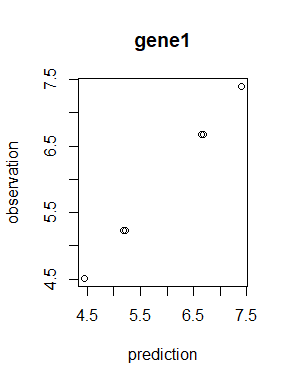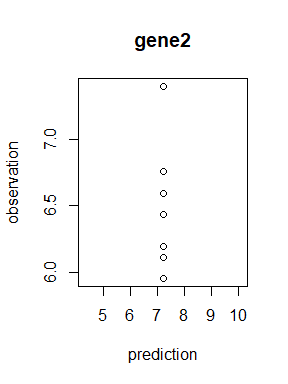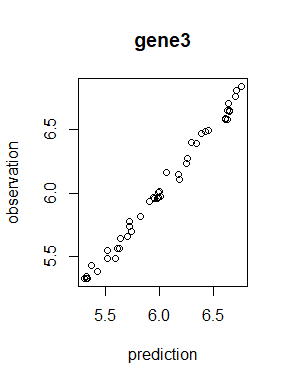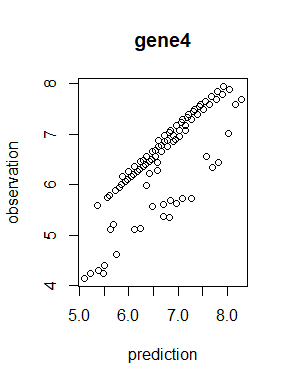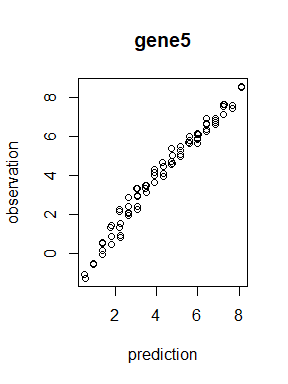## Details of the model

As mentioned above, package FIT predicts gene expression levels using the following equation: $\hat{\boldsymbol s}=\beta_0 + \boldsymbol X \boldsymbol \beta.$ Regression coefficients $$\beta_0$$ and $$\boldsymbol\beta$$ are present as variable coef of the S4 object representing the model whose list is returned by function FIT::train().

models[]$coefs ## intercept coef.age coef.genotype ## 5.92596778 0.00000000 0.00000000 ## coef.clock.cos coef.clock.sin coef.ageClock.cos ## 1.03959868 -0.01421778 0.00000000 ## coef.ageClock.sin coef.env.temperature coef.ageEnv.temperature ## 0.00000000 0.00000000 0.00000000 Here, intercept is $$\beta_0$$ and the remaining elements are those of $$\boldsymbol\beta$$. Design matrix $$\boldsymbol X$$ is constructed as $\boldsymbol X=\left( \boldsymbol d, \boldsymbol n, \boldsymbol c^{cos}, \boldsymbol c^{sin}, \boldsymbol r, \boldsymbol d\circ\boldsymbol c^{cos}, \boldsymbol d\circ\boldsymbol c^{sin}, \boldsymbol d\circ\boldsymbol r \right).$ Here, $$\boldsymbol a\circ\boldsymbol b$$ means an element-wise product of two vectors $$\boldsymbol a$$ and $$\boldsymbol b$$. The plant’s age $$\boldsymbol d$$ is the vector of the numbers of days after transplanting scaled to have the mean of $$0$$ and standard deviation of $$1$$. Each element of vector $$\boldsymbol n$$ indicates a genotype of a smaple. Elements coef.age and coef.genotype in coefs represent regression coefficients of the plant’s age and genotype, respectively. The circadian clock in sample $$j$$ is represented by the cosine and sine curves with a $$24~\mathrm{hr}$$ period as $c^{cos}_j=\frac{\cos\left(2\pi\left(t_j\right)/24\right)}{2},\\ c^{sin}_j=\frac{\sin\left(2\pi\left(t_j-\varphi\right)/24\right)}{2},$ where $$t_j$$ is the time when the sample $$j$$ was obtained. The regression coefficients of these two curves are coef.clock.cos and coef.clock.sin, respectively. The linear combination of these two curves is equal to the cosine curve, that is, $\beta_{cos}c^{cos}_j+\beta_{sin}c^{sin}_j=\sqrt{\beta_{cos}^2+\beta_{sin}^2} \frac{\cos\left(2\pi t_j-\arg\left(\beta_{cos}+i\beta_{sin}\right)\right)}{2}.$ Here, $$\arg\left(\beta_{cos}+i\beta_{sin}\right)$$ is the gene specific phase of the circadian clock. Through training, FIT selects the best environmental factor to explain the variation of gene expression. The selected environmental factor is represented by the variable env of the model object. The response to environmental stimuli is the cumulative sum of an environmental stimulus during a given period $$p$$, that is, $r=\sum^t_{T=t-p}g(T)f(w_T-\theta).$ Here $$g(T)$$ is a gate function that represents a diurnal change in a sensitivity to environmental stimuli. $$f(\cdot)$$ is a response function that characterizes the type of response to stimuli. Parameters $$w_T$$ and $$\theta$$ represent the value of a meteorological parameter at time $$T$$ and the response threshold, respectively. The parameters related to the response are contained in the model object as the variable params. models[]$params
##     env.temperature.period  env.temperature.amplitude
##                 72.5623066                 -0.8073827
##  env.temperature.threshold     gate.temperature.phase
##                 -0.7641115               1378.3190240
## gate.temperature.amplitude gate.temperature.threshold
##                144.5374218                  0.4614072

Here, env.temperature.period and env.temperature.threshold are period $$p$$ and threshold $$\theta$$, respectively. The term between two “.” in the names represents env, that is, which environmental factor the model responds to. For instance, the model in question responds to temperature.

The gate function is defined as $g(T)= \frac{ \tanh\left( \exp\left(\gamma_g\right) \left( \cos\left( 2\pi\left(T-\psi\right)/24 \right) -\theta_g \right) \right) -\tanh\left( \exp\left(\gamma_g\right)\left(-1-\theta_g\right) \right) } { \tanh\left( \exp\left(\gamma_g\right)\left(1-\theta_g\right) \right) -\tanh\left( \exp\left(\gamma_g\right)\left(-1-\theta_g\right) \right) },$ where $$\psi_g$$ determines at what time of day the gene is most sensitive to environmental stimuli, and $$\gamma_g$$ and $$\theta_g$$ control the shape and the opening length of the gate, respectively. A smaller value of $$\theta_g$$ results in longer time of opening of the gate. The shape of this function becomes approximately rectangular with a smaller value of $$\gamma_g$$ and becomes a cosine curve with a larger value of $$\gamma_g$$. In params, $$\psi_g$$, $$\gamma_g$$, and $$\theta_g$$ are present as gate.*.phase, gate.*.amplitude, and gate.*.threshold, respectively (“*" is an environmental factor).

We can consider two types of the response functions. One type responds to environmental stimuli if and only if it is greater than the threshold. On the other hand, the other type responds to stimuli smaller than the threshold. These two types of the response functions are defined as $f_{p}(x)=\max\left(0, \tanh\left(\exp\left(\gamma_f\right)x\right)\right)\sqrt{\exp\left(-2\gamma_f\right)+1},\\ f_{n}(x)=\max\left(0, \tanh\left(-\exp\left(\gamma_f\right)x\right)\right)\sqrt{\exp\left(-2\gamma_f\right)+1}.$ Here, $$f_{p}(x)$$ is the former type, and $$f_{n}(x)$$ is the latter type of the response function. The better type of the response function is chosen at the stage of the optimization of the parameters. It $$f_{p}(x)$$ is chosen, the value of response.type of the model object is $$1$$. Otherwise, it is $$-1$$. As $$\gamma_f$$ approaches minus infinity, the response approaches a dose-dependent response. Conversely, the response approaches a dose-independent response in the limit $$\gamma_f\rightarrow\infty$$. Element params\$env.*.amplitude represents $$\gamma_f$$.

During training, FIT normalizes the values of meteorological data of each environmental factor to have the mean of $$0$$ and standard deviation of $$1$$ as the plant’s age. The mean values and standard deviations of raw data are held in input.mean and input.sd of the model object.